# Hall Effect Applications of Hall Effect

Whenever we place a current carrying conductor in a magnetic field, there is a deflection of the charge carriers due influence of magnetic field in the conductor body. We call this typical phenomenon as Hall effect. Edwin Hall in 1879 had first observed the phenomenon, and hence we call this as Hall effect.

Mainly Lorentz force is responsible for Hall effect. All of we know that when we place a current carrying conductor inside a magnetic field, the conductor experiences a mechanical force to a direction depending upon the direction of magnetic field and the direction of current in the conductor. The electric current means a flow of charge. In metal it is entirely due to the flow of electrons, in semiconductor, it is due to flow of free electrons as well as holes. In semiconductor, holes move in the direction of conventional current and free electrons move in the opposite of the direction of conventional current. As the electrons have charge, they experience a force while flowing through a conductor placed inside a magnetic field. Due to this force, the electrons get diverted towards one side of the conducted during flowing. As the following charges get shifted to one side of the conductor, there may be a tiny potential difference appeared across the cross-section of the conductor. We call this entire phenomenon as hall effect.

Let us take a block of metal as shown below. We show here it as transparent for better understanding.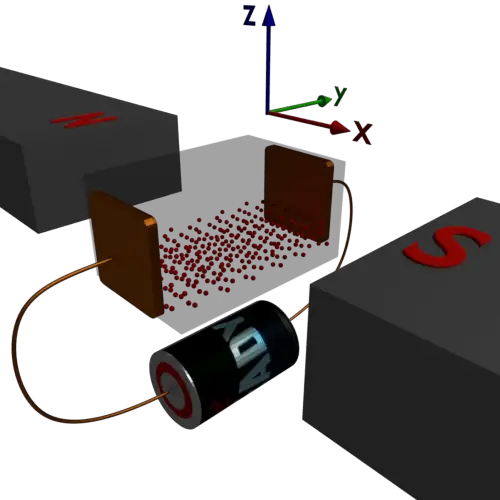After applying a voltage across it current, “I” starts flowing from left to right that is along the x-direction. We place the metal block in a magnetic field of density “B”. The direction of the magnetic field along the y-axis. Now by Fleming’s Left-Hand Rule, the charge carriers will experience a force depending on both – direction of current and direction of magnetic field. As per Fleming’s rule, the conduction electrons will deflect towards the bottom of the block. As a result, there will be a change in concentration of electrons at upper and lower portion of the block. Consequently, a tiny potential difference appears across the block along the z-axis. The electric field created due to this shifting of electrons also opposes the shifting of electrons towards the surface of the metal block. Hence there may be two forces acting on the charge carriers.

1. The force due to Hall effect
2. The force due to created electric field

These forces are opposite to each other. After the establishment of the certain electric field due to Hall effect the system becomes in equilibrium. At that condition the force acting on the charge carriers (conduction electrons) due to the established electric field and due to Hall effect become same, and opposite. Hence there would not be any further shifting of electrons towards the surface of the block and the system become in an equilibrium condition.
Let us concentrate on a single charge carrier moving through a conductor placed inside a magnetic field.
The charge of the charge carrier is e (say)
The magnetic flux density of the field is B (say).
Now we can write the magnetic force acting on the current carrying conductor of active length L and current I as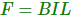When the direction of B and I are perpendicular to each other.

We can rewrite the above equation as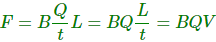Where V is the velocity of the charge passing through the conductor.

From the above equation, we can write the force acting on a single charge carrier as,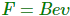Where v is the drift velocity of the charge carriers.
Now we can write the field created due to Hall effect as, EH.
Hence, we can write the force acting on the charge carrier due to the field as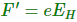Now at equilibrium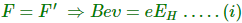Now we consider N is charge carrier concentration.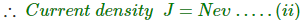From equation 1 and 2, we can write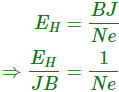We call the term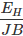as the Coefficient of Hall Effect or simply Hall Coefficient. We define Hall Coefficient as the Hall field per unit magnetic field density per unit current density.

## Application of Hall Effect

Hall effect is a very useful phenomenon and helps to
Determine the Type of Semiconductor
By knowing the direction of the Hall Voltage, one can determine that the given sample is whether n-type semiconductor or p-type semiconductor. This is because Hall coefficient is negative for n-type semiconductor while the same is positive in the case of p-type semiconductor.

Calculate the Carrier Concentration
The expressions for the carrier concentrations of electrons (n) and holes (p) in terms of Hall coefficient are given by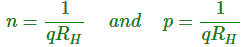Determine the Mobility (Hall Mobility)
Mobility expression for the electrons (μn) and the holes (μp), expressed in terms of Hall coefficient is given by,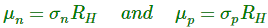Where, σn and σp represent the conductivity due to the electrons and the holes, respectively.

Measure Magnetic Flux Density
This equation can be readily deduced from the equation of Hall voltage and is given by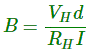Further, there are many commercially available types of equipment based on the principle of Hall effect including Hall-effect sensors and Hall-effect probes.

Want To Learn Faster? 🎓
Get electrical articles delivered to your inbox every week.
No credit card required—it’s 100% free.